### 100 Dollar Note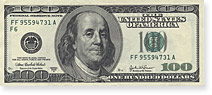W x H = 155.81 x 66.42 mm

### 50 Dollar Note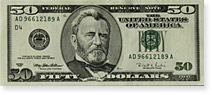W x H = 155.81 x 66.42 mm

### 20 Dollar Note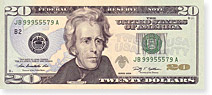W x H = 155.81 x 66.42 mm

### 10 Dollar Note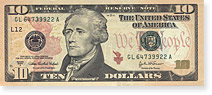W x H = 155.81 x 66.42 mm

### 5 Dollar Note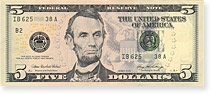W x H = 155.81 x 66.42 mm

### 2 Dollar Note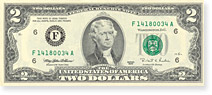W x H = 155.81 x 66.42 mm

### 1 Dollar Note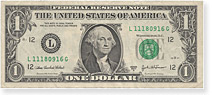W x H = 155.81 x 66.42 mm

Essential cookies are necessary for the operation of the website, they cannot be deactivated. Select the other cookie settings here:

- Marketing cookies are not active
enabled | disable

enable | disable
» Homepage

### 1 Dollar Coin (Buck)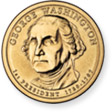Weight = 8.1 g,
Diameter = 26.5 mm
Thickness = 2 mm

### 50 Cent Coin (Half)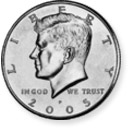Weight = 11.34 g,
Diameter = 30.61 mm
Thickness = 2.15 mm

### 25 Cent Coin (Quarter)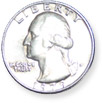Weight = 5.67 g,
Diameter = 24.26 mm
Thickness = 1.75 mm

### 10 Cent Coin (Dime)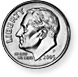Weight = 2.27 g,
Diameter = 17.91 mm
Thickness = 1.35 mm

### 5 Cent Coin (Nickel)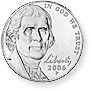Weight = 5 g,
Diameter = 21.21 mm
Thickness = 1.95 mm

### 1 Cent Coin (Penny)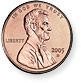Weight = 2.5 g,
Diameter = 19.05 mm
Thickness = 1.55 mm